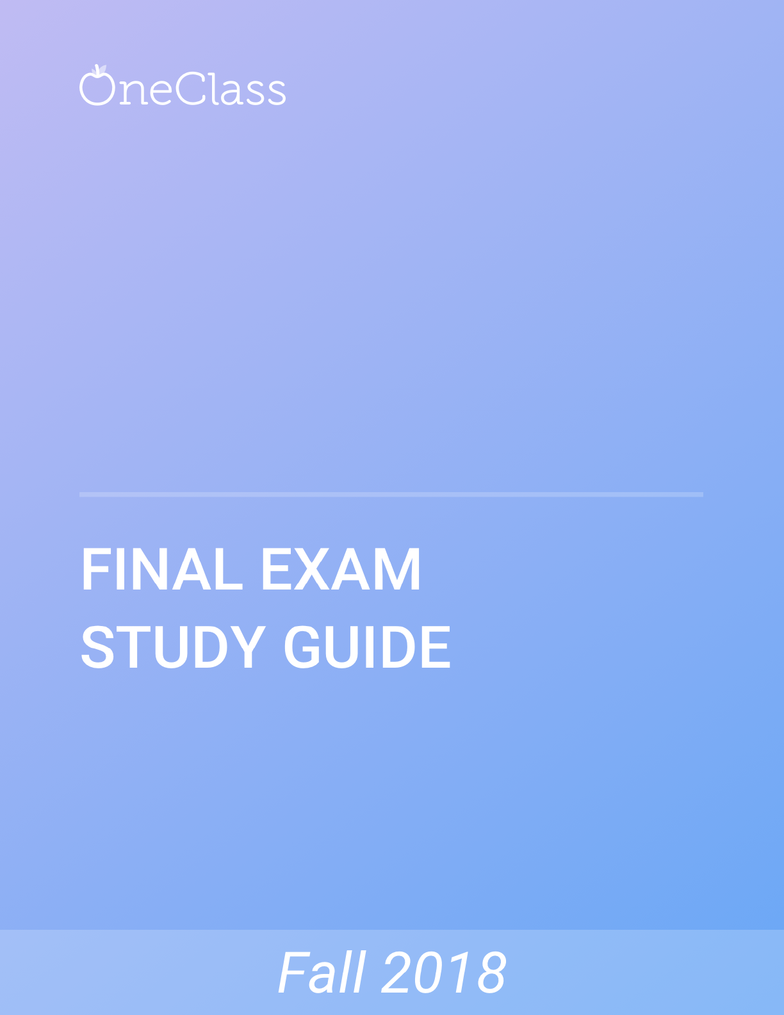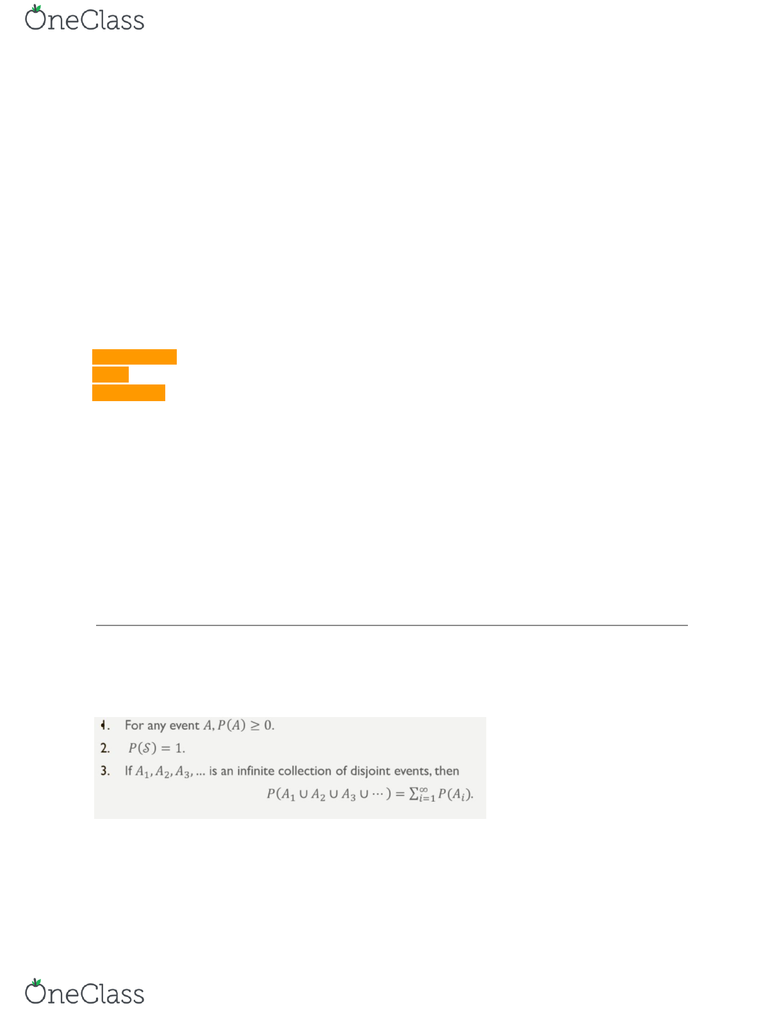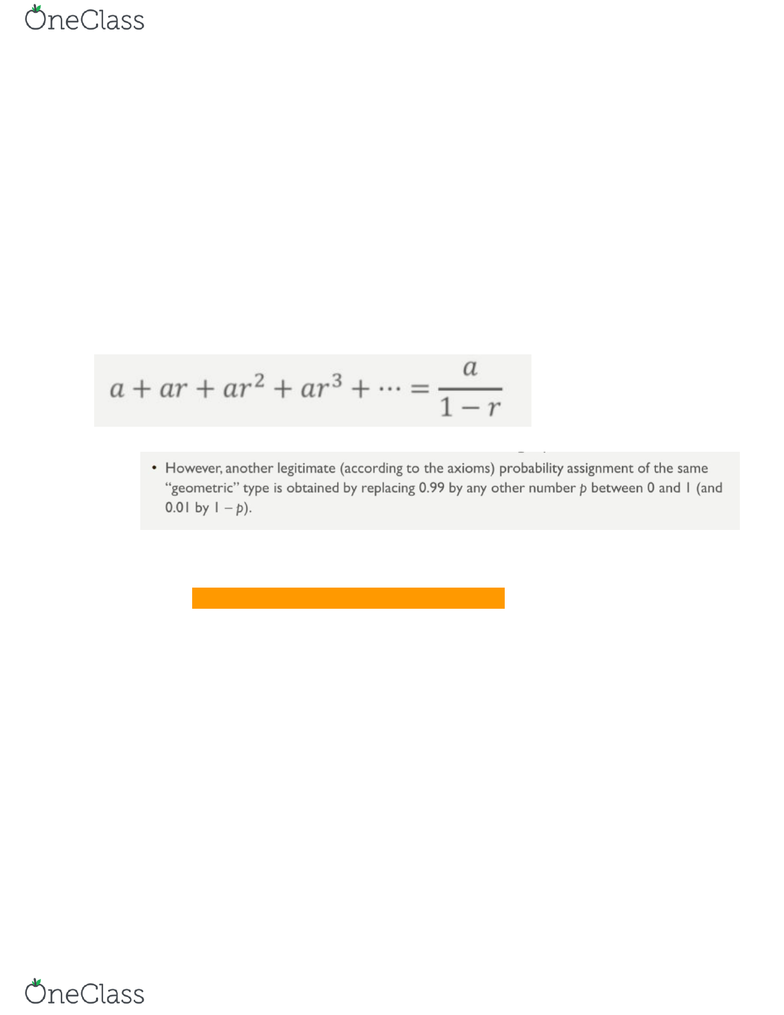# STA 3381 Study Guide - Comprehensive Final Exam Guide - Test Cricket, Old Testament, Factorial

187 views56 pages
School
Course
ProfessorSTA 3381
Unlock document

This preview shows pages 1-3 of the document.
Unlock all 56 pages and 3 million more documents.Unlock document

This preview shows pages 1-3 of the document.
Unlock all 56 pages and 3 million more documents.2.1
Experiment- anything that can have an uncertain outcome
Sample space - set of all possible outcomes of the experiment
Simple experiment only has 2 outcomes
Weld is either defective of not defective.
Events - any collection (subset) of outcomes contained in the sample space S.
Simple if contains one outcome, compound if it contains more than one outcome
Size of the sample space is the number of simple events.
Ex: experiment to find which outcomes will have at least one defective weld → for set S={NNN, DNN,
NDN, NND, NDD, DND, DDN DDD} only the ones that include a D.
Complements of an event A’ is the complement of A
Union of two events A B
Intersection of two events A C
( A C)’ → do what’s inside the parhenthesis first!
(A C) B’
No enclosures required if doing repetitive intersections and unions
If there are no outcomes in common (mutually exclusive or disjoint event), theree are no values that fall
in the set, so it is a null set. Denoted by
A B = (A and B are mutually exclusive or disjoint events) ⊘
visual representation
Rectangle includes the whole sample space
An event is represented by a circle
2.2
Three basic rules (axioms) of probability
* Even if I had A1 A2 A3, (FINITE), the rule still applies.
If something has to happen, it has probability 1. A certain event.
find more resources at oneclass.com
find more resources at oneclass.com
Unlock document

This preview shows pages 1-3 of the document.
Unlock all 56 pages and 3 million more documents.If a set has nothing in it, there is no probability of occurance
P( =0)
Probability will always equal 1 if it can’t happen at the same time.
Any probabililty we have can only be 0 and 1.
Battery example
If multiple events repeated over and over again, then just multiply the events.
Probability of success on first try: P(E1)=0.99
Probabililty of success on second try: P(E2)=(0.01)(0.99)
P(E3)=(0.01)^2*(0.99)
P(Ei)=0.01^(i-1)*0.99
a is probabilty of sucess, r is failure
n(A) denotes the number of replications on which A does occur
The ratio n(A)/n is called the relative frequency
of occurance of the event A in
the sequence of n replications
Package example
For example, Package #3
n(A)/n = ⅔=0.667
Long term probability, as the number of replications increase, the frequency stabilizes. Converge to a
specific number. The number it converges to is limiting (or long-run) frequency
of the event A.
It depends on how long it takes to converge.
As the number increases, the long rul value is going to become more accuracy
Package arrival example
60 percent is the limiting frequency, so overall 60% of the packages arrive on first day. Not exactly 60%,
but we can expect that to happen.
For any event A, P(A)+P(A’)=1, from which P(A)=1-P(A’).
For batter ex, P(success)=1-P(failure)
0.99=1-P(failure)
find more resources at oneclass.com
find more resources at oneclass.com
Unlock document

This preview shows pages 1-3 of the document.
Unlock all 56 pages and 3 million more documents.

## Get access